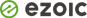The AttributeError: ‘numpy.ndarray’ object has no attribute ‘index’ occurs when you attempt to use the `index()` method on a NumPy array that does not have any index attribute to use.

In this article, we will see what exactly ‘numpy.ndarray’ object has no attribute ‘index’ means and how to resolve this with examples.

## What is AttributeError: ‘numpy.ndarray’ object has no attribute ‘index’?

We get an object has no attribute index error when we try to index the NumPy array with the index function.

Let us take a simple example to reproduce this issue. In our below example, we have declared the NumPy array, and we are trying to get the index of the largest number in an array.

We can find the largest number in the NumPy array using the `max()` function, and to find an index position, we are using the index() method.

When we run the below program, we get AttributeError: ‘numpy.ndarray’ object has no attribute ‘index’.

``````# import numpy library
import numpy as np

# create NumPy array
my_array = np.array([3, 5, 2, 1, 6, 9, 8, 4])

# find the largest number in numpy array
largest_num = np.max(my_array)
print("Largest number in array is", largest_num)

# find the index of largest number
my_array.index(largest_num)
``````

Output

``````Largest number in array is 9
Traceback (most recent call last):
File "C:PersonalIJSCodeprogram.py", line 13, in <module>
my_array.index(largest_num)
AttributeError: 'numpy.ndarray' object has no attribute 'index'``````

## How to fix AttributeError: ‘numpy.ndarray’ object has no attribute ‘index’?

We cannot apply an `index()` function to the NumPy array. The index function works only on the normal Python list.

If we want to find the index position of an element in a NumPy array, we can leverage the `where()` function.

Syntax:

`Numpy.where(array_name==element_of_array)`

The `where()` method returns the indexes of a specified element in a NumPy array as shown in below examples.

### Example 1: Get the index position of an element in the NumPy array

``````# import numpy library
import numpy as np

# create NumPy array
my_array = np.array([3, 5, 2, 1, 6, 9, 8, 4])

# find the largest number in numpy array
largest_num = np.max(my_array)
print("Largest number in array is", largest_num)

# print the index of largest number
print(np.where(my_array == largest_num))
``````

Output

``````Largest number in array is 9
(array(, dtype=int64),)``````

The indexes in the array start from 0, in the above example, the largest element is 9, and it is at the index position 5 in our example.

### Example 2: Get all the index positions of an element in the NumPy array

In this example, the largest element is 9, but it appears more than once in an array. Hence the `where()` function will return all the index positions of the largest element.

In the below example the index positions are 2 and 6 for the largest element 9.

``````# import numpy library
import numpy as np

# create NumPy array
my_array = np.array([3, 5, 9, 2, 1, 6, 9, 8, 4])

# find the largest number in numpy array
largest_num = np.max(my_array)
print("Largest number in array is", largest_num)

# print the indexes of largest number
print(np.where(my_array == largest_num))
``````

Output

``````Largest number in array is 9
(array([2, 6], dtype=int64),)``````

### Example 3: Returns an empty array if the element is not found in the NumPy array

If we pass an element that is not present in an array, the `where()` function will return an empty array. Here the value 10 is not present, and hence it returns an empty array.

``````# import numpy library
import numpy as np

# create NumPy array
my_array = np.array([3, 5, 9, 2, 1, 6, 9, 8, 4])

# print the index of value 10
print(np.where(my_array == 10))
``````

Output

``(array([], dtype=int64),)``

## Conclusion

The index function works only on the normal Python list. If we try to apply the `index()` method on the NumPy array to find the element position, we get AttributeError: ‘numpy.ndarray’ object has no attribute ‘index’ .

We can solve this error by using the `where()` function to get the indexes of the element in a NumPy array.